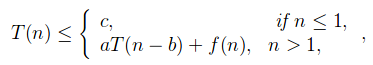# Master Theorem For Subtract and Conquer Recurrences

• Difficulty Level : Medium
• Last Updated : 31 May, 2021

Master theorem is used to determine the Big – O upper bound on functions which possess recurrence, i.e which can be broken into sub problems.
Master Theorem For Subtract and Conquer Recurrences
Let T(n) be a function defined on positive n as shown below:for some constants c, a>0, b>0, k>=0 and function f(n). If f(n) is O(nk), then
1. If a<1 then T(n) = O(nk
2. If a=1 then T(n) = O(nk+1
3. if a>1 then T(n) = O(nkan/b)
Proof of above theorem( By substitution method ):
From above function, we have:
T(n) = aT(n-b) + f(n)
T(n-b) = aT(n-2b) + f(n-b)
T(n-2b) = aT(n-3b) + f(n-2b)
Now,
T(n-b) = a2T(n-3b) + af(n-2b) + f(n-b)
T(n) = a3T(n-3b) + a2f(n-2b) + af(n-b) + f(n)
T(n) = Σi=0 to n ai f(n-ib) + constant, where f(n-ib) is O(n-ib)
T(n) = O(nk Σi=0 to n/b ai )

Where,
If a<1 then Σi=0 to n/b ai = O(1), T(n) = O(nk)
If a=1 then Σi=0 to n/b ai = O(n), T(n) = O(nk+1
If a>1 then Σi=0 to n/b ai = O(an/b), T(n) = O(nkan/b)
Consider the following program for nth fibonacci number

## C++

 `#include``int` `fib(``int` `n)``{``   ``if` `(n <= 1)``      ``return` `n;``   ``return` `fib(n-1) + fib(n-2);``}`` ` `int` `main ()``{``  ``int` `n = 9;``  ``printf``(``"%d"``, fib(n));``  ``getchar``();``  ``return` `0;``}`

## Python3

 `# Python3 code for the above approach``def` `fib(n):``    ``if` `(n <``=` `1``):``        ``return` `n``    ``return` `fib(n ``-` `1``) ``+` `fib(n ``-` `2``)` `# Driver code``n ``=` `9``print``(fib(n))` `# This code is contributed``# by sahishelangia`

## Java

 `//Java code for above the approach.``class` `clg``{`` ``static` `int` `fib(``int` `n)``{``if` `(n <= ``1``)``    ``return` `n;``return` `fib(n-``1``) + fib(n-``2``);``}``// Driver Code``public` `static` `void` `main (String[] args)``{``int` `n = ``9``;``System.out.println( fib(n));``}``}``// This code is contributed by Mukul Singh.`

## C#

 `// C# code for above the approach.``using` `System;``    ` `class` `GFG``{``    ``static` `int` `fib(``int` `n)``    ``{``        ``if` `(n <= 1)``            ``return` `n;``        ``return` `fib(n - 1) + fib(n - 2);``    ``}``    ` `    ``// Driver Code``    ``public` `static` `void` `Main(String[] args)``    ``{``        ``int` `n = 9;``        ``Console.WriteLine(fib(n));``    ``}``}` `// This code has been contributed``// by Rajput-Ji`

## PHP

 ``

## Javascript

 ``

Output

`34`

Time complexity Analysis:
The recursive function can be defined as, T(n) = T(n-1) + T(n-2)

• For Worst Case, Let T(n-1) ≈ T(n-2)
T(n) = 2T(n-1) + c
where,f(n) = O(1)
∴ k=0, a=2, b=1;
T(n) = O(n02n/1
= O(2n

• For Best Case, Let T(n-2) ≈ T(n-1)
T(n) = 2T(n-2) + c
where,f(n) = O(1)
∴ k=0, a=2, b=2;
T(n) = O(n02n/2
= O(2n/2

More Examples:

• Example-1
T(n) = 3T(n-1), n>0
= c, n<=0
Sol:a=3, b=1, f(n)=0 so k=0;
Since a>0, T(n) = O(nkan/b
T(n)= O(n03n/1
T(n)= 3n

• Example-2
T(n) = T(n-1) + n(n-1), if n>=2
= 1, if n=1
Sol:a=1, b=1, f(n)=n(n-1) so k=2;
Since a=1, T(n) = O(nk+1
T(n)= O(n2+1
T(n)= O(n3

• Example-3
T(n) = 2T(n-1) – 1, if n>0
= 1, if n<=0
Sol: This recurrence can’t be solved using above method
since function is not of form T(n) = aT(n-b) + f(n)

This article is contributed by Yash Singla. If you like GeeksforGeeks and would like to contribute, you can also write an article using write.geeksforgeeks.org or mail your article to contribute@geeksforgeeks.org. See your article appearing on the GeeksforGeeks main page and help other Geeks.
Please write comments if you find anything incorrect, or you want to share more information about the topic discussed above.

My Personal Notes arrow_drop_up